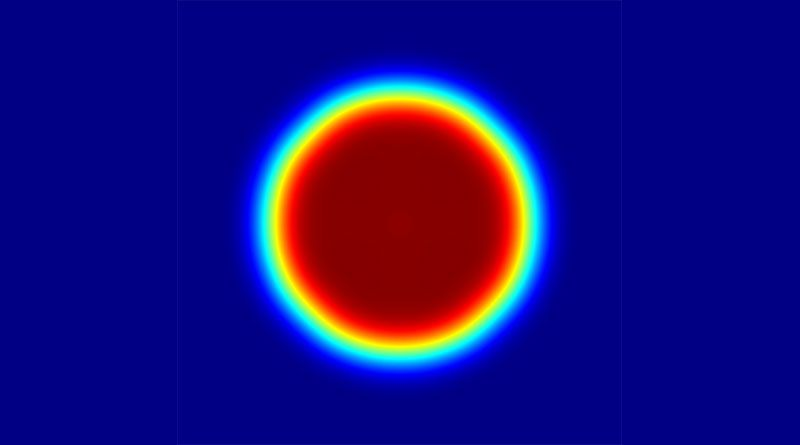# Diffractive Optics lensesDiffractive Optical lenses are a type of diffractive optical elements that can perform the same function of a conventional optical lens. In a regular lens, a beam of light is brought to a focus by bending the light rays in a continuous way across the element’s clear aperture. This bending of rays can be fully analysed in terms of geometrical optics. In contrast, a diffractive optical lens works by exploiting the wave nature of light. In other words, the focused spot of a diffractive optical lens is said to be formed by an interference effect.

To design a diffractive optical lens of a certain focal length distance, the first step is to calculate an equivalent radius of curvature on a given glass or plastic substrate that will produce the desired focal length. This process results in a regular lens. The next step is to partition or sample the resulting curvature into smaller areas.  Thereafter, each area is collapsed, that is, their starting point along the optical axis is the same for all areas. The resulting structure is no longer continuous, but still does contain all the information about the original lens curvature. At this stage, the component is referred to as a Fresnel lens. The next step needed to arrive at a proper diffractive optical lens is to quantize the curvature on each patch according to some predetermined phase steps.  The number of steps will depend on the manufacturing constraints, but it is usually in powers of two.

One important advantage of diffractive optical lenses is that they can be much lighter and thinner, for obvious reasons. Considering this crucial aspect, a diffractive optical lens can be very useful in those applications in which space or weight, or even both, are critical parameters of the system in question. Another advantage of diffractive optical lenses is that they do not suffer from a type of aberration referred to as field curvature. This type of error refers to a defocus error that grows as a point image moves off-axis.

Diffractive optics lenses are meant to work with monochromatic light. The position of the focal plane varies considerably among wavelengths of the visible spectrum, for example. Nevertheless, given that the resulting dispersion is in the opposite direction of what would occur in a conventional lens system, a diffractive optical lens can be used to balance out the overall dispersion on a multi-element optical system.